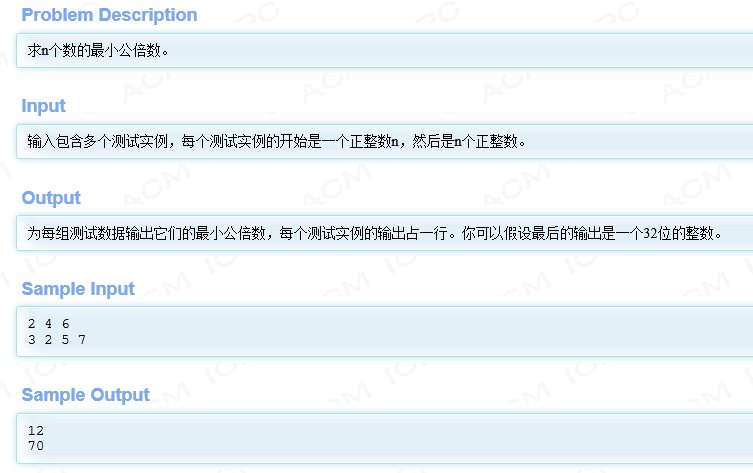【新人求问】c语言，求最最小公倍数``````#include<stdio.h>
int main(int argc, char* argv[])
{
int n;  //一组中数的个数
int s;
int i;
int x;  //用于存储一组数中的最大数
while (scanf("%d",&n)!=EOF)
{
x = 0
for (i = 0; i < n; i++)
{
scanf("%d",&s[i]);
if (s[i]>x)
x = s[i];
}
while(1)
{
for (i = 0; i < n; i++)
{
if (x%s[i]!=0)
break;
}
if(i >= n)
break;
x++;
}
printf("%d\n",x);
}
return 0;
}
``````

6个回答

http://ideone.com/rujbTf

x = 0 这里少一个分号

32位的整数需不需要修改下输出的整数范围，修改成long long int 比较稳妥，没有看具体代码，，zuosidelord 哎呀呀，宝宝想撤销回答，果然需要先看看代码，，，，，，，，，，，，，，，楼主请无视我的回答吧